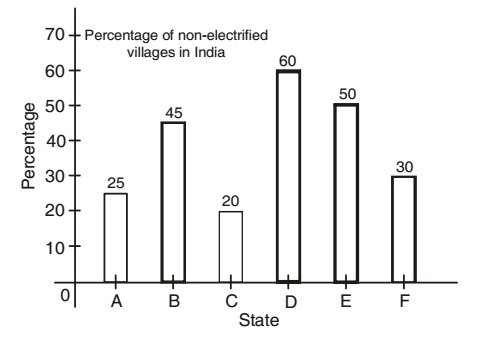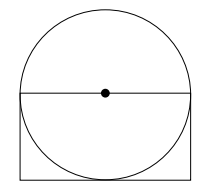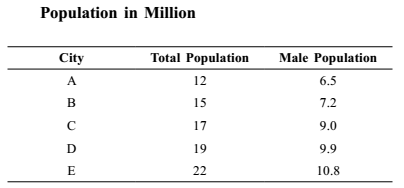## Bar graph

#### Data Interpretation

Direction: Study the graph given below and answer the question asked there under.1. How many states have at last 60% or more electrified villages?

1. In A, C and F states, percentage of non-electrified villages are 25, 20 and 30 per cent respectively.

##### Correct Option: A

In A, C and F states, percentage of non-electrified villages are 25, 20 and 30 per cent respectively. Hence, percentages of electrified villages for these states are 75, 80 and 70 percent respectively.

1. In which of the following states percentage of electrified villages is double the percentage of electrified villages in state (D)?

1. Percentage of electrified villages in state D = 100 - 60
Percentage of electrified villages in state C = 100 - 20

##### Correct Option: A

Percentage of electrified villages in state D = 100 - 60 = 40
Percentage of electrified villages in state C = 100 - 20 = 80
Hence, the percentage of electrified villages in the state C is double the percentage of electrified villages in state D.

1. What is the precentage of electrified villages in the state (A)?

1. Percentage of electrified villages in the state A = 100 - 25 .

##### Correct Option: D

Percentage of electrified villages in the state A = 100 - 25 = 75%

Direction: Study the information given in each of these questions and then answer the questions.

1. The area of the circle is 616 cm2. What is the area of the rectangle?1. ∵ Area of the circle = πr2
Required Area of the rectangle = Length x Breadth

##### Correct Option: C

given :- Area of the circle = 616
⇒ πr2 = 616
Put the value of π = 22/7 in above equation.
we will get,
⇒ r2 = 196
⇒ r = 14 cm
Length of the rectangle = Diameter of the circle = 2r
∴ Area of the rectangle = Length x Breadth
∴ Area of the rectangle = 28 × 14 = 392 cm2

1. What is the average female population in million?1.  Average female population = sum of total numbers of females total number of cities

##### Correct Option: A

From above given table , we can see that
The total population of all cities = 12 + 15 + 17 + 19 + 22 = 85 million
Total males in all cities = 6.5 + 7.2 + 9.0 + 9.9 + 10.8 = 43.4 million
Total females = 85 – 43.4 = 41.6 million

 Average female population = sum of total numbers of females total number of cities

 = 41.6 = 8.32 million 5